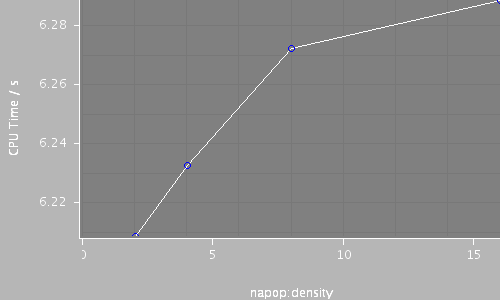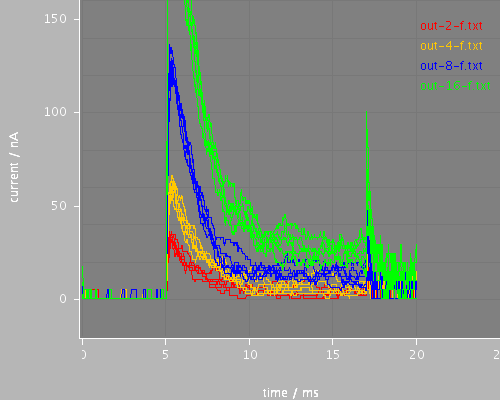## p1-f1-mean-variance (run.xml)

Voltage clamp with stochastic channels. There are four cases varying in the density of channels in the model (from 2 or to 16 per square micron). Each case is run 400 times of which six are shown in the default plots. The mean variance analysis operates on the 5-10 ms time window and shows the variance against the mean current. The form should be a parabola, with the slope at the origin giving the single channel conductance and the intercept on the X axis the total number of channels.

Total CPU time 10.82 seconds; at 12:47:50 Thu 24 Sep 2009

 Compartments Stochasticchannels / cpmts Continuouschannels / cpmts Non Gatedchannels / cpmts time/ms timestep/ms napop:density CPU Time / s 1 25 / 1 0 / 0 0 / 0 20.0 0.01 2 2.43 1 50 / 1 0 / 0 0 / 0 20.0 0.01 4 3.18 1 101 / 1 0 / 0 0 / 0 20.0 0.01 8 2.45 1 201 / 1 0 / 0 0 / 0 20.0 0.01 16 2.76#### Morphology: cell### Predefined views

#### whole#### mvwhole## All files

 Model Preprocessed Outupt data Reference data etc run.xml cell.xml membrane.xml environment.xml recording.xml na1.xml out-2.ppp out-4.ppp out-8.ppp out-16.ppp log.txt out-2.sum out-4.sum out-8.sum out-16.sum out-2.dat out-2.txt out-4.dat out-4.txt out-8.dat out-8.txt out-16.dat out-16.txt ref-continuous.txt

## Model

Archive file of the complete model: p1-f1-mean-variance.jar

### run.xml

```<PSICSRun timeStep="0.01ms" runTime="20ms" startPotential="-65mV"
morphology="cell"
environment="environment"
properties="membrane"
access="recording"
stochThreshold="1000"
repeats="400">
<StructureDiscretization baseElementSize="3um"/>

<RunSet vary="napop:density" values="[2, 4, 8, 16]per_um2"
filepattern="out-\$">
</RunSet>

Voltage clamp with stochastic channels. There are four cases varying in
the density of channels in the model (from 2 or
to 16 per square micron).  Each case is run 400 times of which six are
shown in the default plots. The mean variance analysis operates on the
5-10 ms time window and shows the variance against the mean current. The form
should be a parabola, with the slope at the origin giving the single channel
conductance and the intercept on the X axis the total number of channels.

<ViewConfig>
<LineGraph width="500" height="400">
<XAxis min="0" max="250" label="time / ms"/>
<YAxis min="-80" max="60" label="current / nA"/>

<LineSet file="out-2.txt" color="red" maxshow="6"/>
<LineSet file="out-4.txt" color="orange" maxshow="6"/>
<LineSet file="out-8.txt" color="blue" maxshow="6"/>
<LineSet file="out-16.txt" color="green" maxshow="6"/>

<View id="whole" xmin="0." xmax="25." ymin="-160." ymax="20."/>
</LineGraph>

<LineGraph>
<XAxis label="mean current, nA"/>
<YAxis label="current variance, nA^2"/>
<MeanVariance file="out-2.txt" tmin="5" tmax="10" binSize="1" color="red"/>
<MeanVariance file="out-4.txt" tmin="5" tmax="10" binSize="2" color="orange"/>
<MeanVariance file="out-8.txt" tmin="5" tmax="10" binSize="10" color="blue"/>
<MeanVariance file="out-16.txt" tmin="5" tmax="10" binSize="20" color="green"/>

<View id="mvwhole" xmin="0" xmax="250" ymin = "0." ymax="200"/>
</LineGraph>

</ViewConfig>

</PSICSRun>
```

### cell.xml

```<CellMorphology id="cell">

<Point id="p0" x="0" y="0" z="0" r="1."/>

</CellMorphology>
```

### membrane.xml

```<CellProperties id="membrane"
cytoplasmResistivity="100ohm_cm"
membraneCapacitance="1.e-8uF_per_um2">

<ChannelPopulation id="napop" channel="na1" density="16per_um2"/>

</CellProperties>
```

### environment.xml

```<CellEnvironment id="environment">
<Ion id="Na" name="Sodium" reversalPotential="80mV"/>
<Ion id="K" name="Potassium" reversalPotential="-80mV"/>
</CellEnvironment>
```

### recording.xml

```<Access id="recording" saveInterval="0.05ms">

<VoltageClamp at="p0" lineColor="red" hold="-80.0mV">
<VoltagePulse id="vstep" start="5ms" to="0mV"
duration="12ms"/>
</VoltageClamp>

</Access>
```

### na1.xml

```<KSChannel id="na1" gSingle="30pS" permeantIon="Na">
<ClosedState id="c1"/>
<OpenState id="o1"/>
<ClosedState id="c2"/>

<VHalfTransition from="c1" to="o1" vHalf = "-35mV" z="4.5e" gamma="0.8" tau="0.55ms" tauMin="0.1ms"/>

<VHalfTransition from="o1" to="c2" vHalf = "-70mV" z="1.1e" gamma="0.90" tau="12.0ms" tauMin="1.5ms"/>

</KSChannel>
```